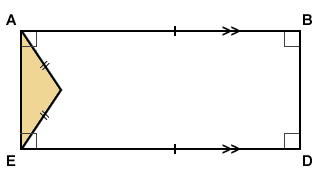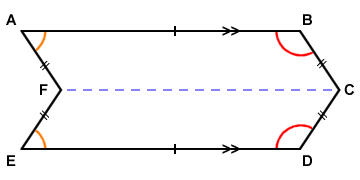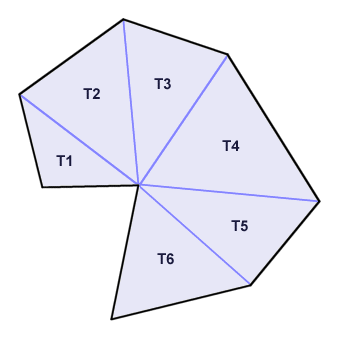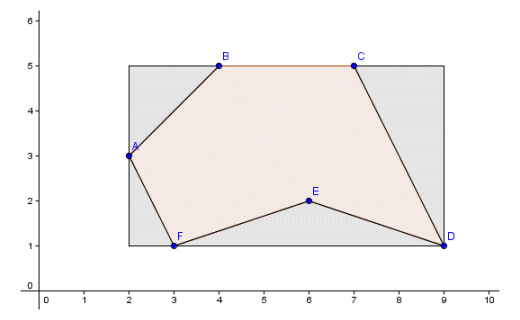# Irregular Polygons

## Overview

If you have looked at the page entitled "Regular Polygons", you may recall that a polygon is a closed, two dimensional figure with multiple (i.e. three or more) straight sides. Since a regular polygon is one in which all of the sides have the same length, and all of the internal angles are of the same magnitude, it follows that an irregular polygon is one that does not meet these criteria (i.e. it is neither equilateral nor equiangular). Like regular polygons, irregular polygons may be simple (i.e. a convex or concave figure in which the sides form a boundary around a single enclosed space, and no internal angle exceeds one hundred and eighty degrees) or complex (two or more sides will intersect one another). We present below some examples of irregular polygons.Examples of irregular polygons

One thing to notice here is that the set of irregular polygons, as well as being infinitely large, includes a number of shapes you may already be familiar with. Shape (a), for example, is a rectangle (and therefore by definition also a parallelogram and a quadrilateral). It is irregular by virtue of the fact that, although opposite sides are equal in length, adjacent sides are not. Shape (b) is an isosceles triangle, and is irregular because only two sides are equal, and only two angles are equal. Shape (c) is both a parallelogram and a quadrilateral. It is irregular because adjacent sides are not equal, and adjacent angles are not equal. Shape (d) is a trapezium and a quadrilateral. It has two equal sides and two pairs of equal angles, but is clearly irregular.

The remaining shapes do not really have specific names. Shape (e) is a complex quadrilateral in which no two sides are equal, and no two angles are equal. Shape (f) is technically a hexagon (because it has six sides). It is however both irregular, because it has sides of different lengths and angles of different magnitudes, and concave, because one of its internal angles is greater than one hundred and eighty degrees ( > 180° ). By the same token, shape (g) is technically a pentagon, since it has five sides, but no two sides are the same length and only two of the five internal angles are the same. Like shape (f), shape (h) is also a concave hexagon, but in this case none of the sides is equal, none of the angles are equal, and no two sides are parallel.

## Finding the area of an irregular polygon

As with any kind of polygon, finding the perimeter is a relatively trivial exercise. Providing you know the length of each side, you can simply add the lengths together to find the perimeter. If the lengths of some or all of the sides are unknown, you will first need to find the length of each side by measuring it. Finding the area is more complicated. In the case of irregular polygons that are either triangles or quadrilaterals, the methods that may be used are described in the relevant pages. For irregular polygons that have more than four sides, other methods must be used. The exact method used in any given case will depend on the kind of irregular polygon we are dealing with. Consider the irregular hexagon illustrated below, for example.An irregular hexagon

In this case, we know that the line segments AB and DE are equal in length, as are the line segments BC, CD, EF and FA. We also know that the line segments AB and DE are parallel. From this information, we can deduce that angles ABC and CDE are equal, and that angles DEF and FAB are equal. We can also deduce that angles ABC and FAB are supplementary (add up to one hundred and eighty degrees) as are angles CDE and DEF. It can also be shown that the line segments BC and FA are parallel, as are the line segments CD and EF. From this information, you should be able to see that if we were to take the triangular portion of the shape defined by points B, C and D and move it so that it covered the triangular area defined by points A, E and F, we would be left with a rectangle as shown below.The irregular hexagon becomes a rectangle

We can see that the irregular hexagon ABCDEF is equivalent in area to the rectangle ABCD, so the area of ABCDEF can be found by taking the product of the lengths of line segments AB and BD. If these lengths are known, this is a straightforward calculation. If not, they must first be found by measurement. In cases such as the above, it is often possible to transform the irregular polygon into a shape for which we already have a method of calculating the area, and which has the same area as the original shape. In other cases this is either difficult or not possible. We could, alternatively, have drawn the line segment FC as shown below, effectively breaking the shape into two parallelograms of equal area. We could then find the area of one of the parallelograms and multiply the result by two to find the total area of the shape.The irregular hexagon could be broken down into two parallelograms of equal area

One method that works for finding the area of any irregular polygon (or any regular polygon for that matter) involves breaking the polygon down into triangles, finding the area of each triangle using standard methods, and adding the areas of the individual triangles together (note that in some cases, a shape can be broken down into a combination of triangles and rectangles, but all polygons can be broken down into triangles). The principle is illustrated below.Any polygon may be broken down into a number of triangular areas

The area of the polygon is the sum of the areas of triangles T1, T2, T3, T4, T5 and T6. The main disadvantage of this method is that it will involve selecting one side of each triangle to be the base, constructing the perpendicular line segment from the base to the apex of the triangle (i.e. the angle opposite the base), and measuring the dimensions of each. Once you know the length l of the base and the height h for each triangle it is a straightforward calculation to find the area:

 Area  = l  ×  h 2

There is also a formula that can be used to find the area of a simple convex or concave irregular polygon, even if every side is of a different length and every angle of a different magnitude. The formula can only be used, however, if you know the coordinates of each of the vertices. It also has the disadvantage that it cannot be used for complex polygons (i.e. polygons in which two or more of the sides intersect one another). Consider the following concave irregular polygon:An irregular concave six-sided polygon

The coordinates of the vertices for the irregular six-sided figure shown above are as follows:

• A        2, 3
• B        4, 5
• C        7, 5
• D        9, 1
• E        6, 2
• F        3, 1

The general formula used to find the area of a simple polygon uses the xy coordinates of each vertex of the polygon from the first to the last in clockwise order around the shape as follows:

 Area  = (x1y2 - y1x2) + (x2y3 - y2x3) . . . + (xny1 - ynx1) 2

Applying the formula to our six-sided irregular polygon, we get:

 Area  = (xAyB - yAxB) + (xByC - yBxC) + (xCyD - yCxD) + (xDyE - yDxE) + (xEyF - yExF) + (xFyA - yFxA) 2
 Area  = (2·5 - 3·4) + (4·5 - 5·7) + (7·1 - 5·9) + (9·2 - 1·6) + (6·1 - 2·3) + (3·3 - 1·2) 2
 Area  = (10 - 12) + (20 - 35) + (7 - 45) + (18 - 6) + (6 - 6) + (9 - 2) 2
 Area  = -2 - 15 - 38 + 12 + 0 + 7 2
 Area  = -36 = -18 2

Don't worry if the result turns out to be negative. Simply change the minus sign to a plus sign. As a quick check on the result, you can calculate the area of the bounding rectangle, since the answer you get should always be less than this figure. In this case, the area of the bounding rectangle will be the product of 9 - 2 (i.e. the difference between the maximum and minimum x coordinates) and 5 - 1 (i.e. the difference between the maximum and minimum y coordinates), which gives us 7 × 4 = 28.

As a further check, it may be possible to calculate the areas of the triangles formed between the perimeter of the bounding rectangle and that of the polygon, as is the case here. Three of the triangles so formed in the example we have used are right-angled triangles. For these triangles, we know the lengths of the sides adjacent to the right-angle, which essentially gives us a base length and height. We also know both the base length and the height of the remaining triangle (triangle DEF), even though this triangle does not contain a right-angle.An irregular concave six-sided polygon with its bounding rectangle

The resulting calculation would look something like this:

 Area  =  (7  ×  4)  - (2  ×  2) + (2  ×  4) + (6  ×  1) + (1  ×  2) 2
 Area  =  28  - 20 2
 Area  =  28 - 10  =  18